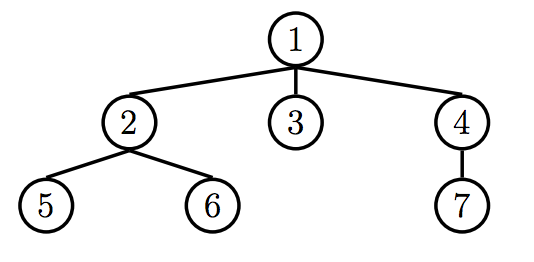# Discussion 13 Guide

## Section 3.2 Question 2

Write a program flatten that given a Tree t, will return a linked list of the elements of t, ordered by level. Entries on the same level should be ordered from left to right. For example, the following tree will return the linked list <1 2 3 4 5 6 7>.This problem actually touches on a concept that is not covered in this course called breadth first search. Take 61B for more info!

We can approach this problem in two ways, using just a list, or traversing the tree twice:

1. To give each node a "level"
2. To put together our linked list, based on the levels we assigned each
node.


### Intuitive Approach

Let’s first try the intuitive approach, which is to traverse t twice. On the first traversal, I will have to keep track of the level of every node. Let’s do this with a dictionary that keeps a list of each node at a level (which will be the key).

levels = {}
define traverser(t, level)
if level not in levels:
levels[level] = []
levels[level].append(t)
[traverser(c, level + 1) for c in t.children]


Now that we have all of our levels (and conveniently, the nodes on the same level will be ordered from left to right because we traverse our children from left to right), we just have to stick it into a Linked List. This part is a little messy.

list_of_nodes = []
for l in range(len(levels)): # dictionaries are unordered!
for n in levels[l]:
list_of_nodes.append(n.entry)

def converter(lst):
if not lst:

converter(list_of_nodes)


Putting it together:

define flatten(t):
levels = {}
define traverser(t, level)
if level not in levels:
levels[level] = []
levels[level].append(t)
[traverser(c, level + 1) for c in t.children]
traverser(t, 0)
list_of_nodes = []
for l in range(len(levels)): # dictionaries are unordered!
for n in levels[l]:
list_of_nodes.append(n.entry)

def converter(lst):
if not lst:

converter(list_of_nodes)


### Approach with List (Queue)

Now let’s go over a way to solve this problem with only a list. The key understanding is that when we traverse a tree, it’s possible to make a list of the nodes in the order that we want.For example, when we start at the root node, we can form a list of nodes (children) that we will want to collect entries from. At the root node 1, we can form a list, or a queue, of nodes to traverse next - the nodes 2, 3, and 4. If this list can be mutated by each function call, we can use this list to figure out which node to look at next.

In the simplest case, our list, after traversing the root node’s children would be [2, 3, 4] (we will traverse the node 2 next). After we traverse the node 2, we would just tack on what we have to explore (2’s children) to the end of our list. So our list of nodes we still need to explore would look like this:

[3, 4, 5, 6] # we explored node 2 already


Now that we have the order of exploration down, we can just return the Linked List of the current entry, and a recursive call to our helper function. Putting that together:

define flatten(t):
def flatten_helper(queue):
if not queue: # queue is empty, we're done exploring
curr = queue.pop(0) # removes the first element of the queue
for c in curr.children:
queue.append(c) # we add future nodes to explore to the end
return flatten_helper([t]) # start it off with the root node


## Section 4 Question 2

Define deep-apply, which takes a nested list and applies a given procedure to every element. deep-apply should return a nested list with the same structure as the input list, but with each element replaced by the result of applying the given procedure to that element. Use the built-in list? procedure to detect whether a value is a list. The procedure map has been defined for you.

(define (map fn lst)
(if (null? lst)
nil
(cons (fn (car lst)) (map fn (cdr lst)))))


This problem is kind of similar to replace_all_deep (Section 1 Q#2). However, with the provided map function, we eliminate having to process element by element.

Let’s look at the case where the list is not deep:

(define (deep-apply fn lst)
(map fn lst))


If we map deep-apply over every element, we don’t need to worry about checking if (car nested-list) is a list, because map is calling deep-apply on each element individually. Thus, we only need to check if nested-list is a list. If it is, we need to map deep-apply over it. If it’s not, we are returning (fn nested-list). This might be a little more intuitive if you replace the argument name nested-list with elem.

We get:

(define (deep-apply fn nested-list)
(if (list? nested-list)
(map (lambda (x) (deep-apply fn x)) nested-list)
(fn nested-list)))

back up